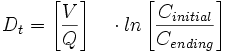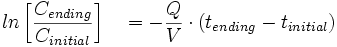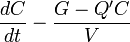# Dilution (equation)

Dilution is a reduction in the concentration of a chemical (gas, vapor, solution). It is the process of reducing the concentration of a solute in solution, usually simply by mixing with more solvent. To dilute a solution means to add more solvent without the addition of more solute. The resulting solution is thoroughly mixed so as to ensure that all parts of the solution are identical.

The same direct relationship applies to gases and vapors diluted in air for example. Although, thorough mixing of gases and vapors may not be as easily accomplished.

For example, if there are 10 grams of salt (the solute) dissolved in 1 liter of water (the solvent), this solution has a certain salt concentration/molarity. If one adds 1 liter of water to this solution the salt concentration is reduced. The diluted solution still contains 10 grams of salt/(0.171 moles of NaCl).

Mathematically this relationship can be shown in the equation:Where:

C1 = Concentration/molarity 1

V1 = Volume 1

C2 = Concentration/molarity 2

V2 = Volume 2

[hide]

//

##  Basic Room Purge Equation

The Basic Room Purge Equation, is used in industrial hygiene. It determines the time required to reduce a known vapor concentration existing in a closed space to a lower vapor concentration. The equation can only be applied when the purged volume of vapor or gas is replaced with “clean” air or gas. For example, the equation can be used to calculate the time required at a certain ventilation rate to reduce a high carbon monoxide concentration in a room.Sometimes the equation is also written as:where tinitial = 0

Dt = Time required; the unit of time used is the same as is used for Q.

V = Air or gas volume of the closed space or room in cubic feet, cubic meters or liters

Q = Ventilation rate into or out of the room in cubic feet per minute, cubic meters per hour or liters per second

Cinitial = Initial concentration of a vapor inside the room measured in ppm

Cending = Final reduced concentration of the vapor inside the room in ppm

##  Dilution Ventilation Equation

The Basic Room Purge Equation can be used only for purge scenarios. In a scenario where a liquid continuously evaporates from a container in a ventilated room, a differential equation has to be used.Where the ventilation rate has been adjusted by a mixing factor K.C = concentration of a gas

G = generation rate

V = room volume

Q’ = adjusted ventilation rate of the volume